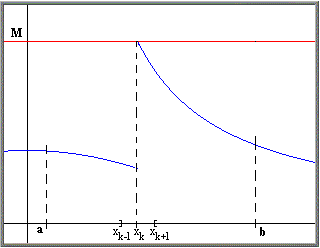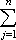#Interactive Real Analysis

Next | Previous | Glossary | Map

## 7.1. Riemann Integral

### Corollary 7.1.17: Riemann Integral of almost Continuous Function

If f is a bounded function defined on a closed, bounded interval [a, b] and f is continuous except at countably many points, then f is Riemann integrable.

### Proof:

We can prove this easily by applying Lebesgue's Theorem, noting that any set with at most countably many points has measure zero.

To prove this is directly, however, is not easy; we will start with a simpler version of this theorem: if f is continuous and bounded over the interval [a, b] except at one point xk, then f is Riemann integrable over [a, b].We know that f is bounded by some number M over the interval [a, b].

Take any> 0 and choose a partition P that includes the point xk such that

| P | </ 12M

Then in particular

|xk+1 - xk-1| </ 6M

We also know that f is uniformly continuous over [a, xk-1] as well as uniformly continuous over [xk+1, b]. Therefore, for our chosenthere exists

• a' such that |f(x) - f(y)| < 1/3/ (b - a) for all x, y inside [a, xk-1] with |x - y| <'
• a'' such that |f(x) - f(y)| < 1/3/ (b - a) for all x, y inside [xk+1, b] with |x - y| <''

Now refine the partition P by adding points on the left side of xk-1 so that the mesh on that side is less than', and by adding points on the right side of xk+1 so that the mesh there is less than''. For simplicity, call that new partition again P. Then we have:

| U(f,P) - L(f,P) ||cj - dj| (xj - xj-1) =For the first term we have:

|c1 - d1| (x1 - x0) + ... + |ck-1 - dk-1| (xk-1 - xk-2)
< 1/3/(b-a) (xk-1 - x0) < 1/3/(b-a) (b - a) = 1/3because of uniform continuity to the left of xk and our choice of the partition. The third term can be estimated similarly:

|ck+2 - dk+2| (xk+2 - xk+1) + ... + |cn - dn| (xn - xn-1)
< 1/3/(b-a) (xn - xk+1) < 1/3/(b-a) (b - a) = 1/3Since f is bounded by M we know that |cj - dj| < 2M for all j so that the middle term can be estimated by:

|ck - dk| (xk - xk-1) + |ck+1 - dk+1| (xk+1 - xk)
< 2M (xk+1 - xk-1) < 2M/ 6M = 1/3Taking everything together we have:

|U(f,P) - L(f,P)| < 1/3+ 1/3+ 1/3=Therefore, by Riemann's Lemma, the function f is Riemann integrable.

Next | Previous | Glossary | Map